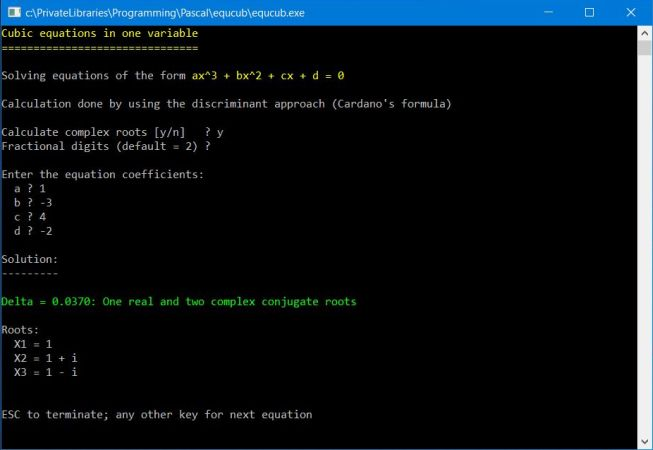# Computing: Free Pascal Programming

 Programming Home Home Contact

## Cubic equations in one variable (equcub).

Description: The program solves cubic equations in one variable (equations of the form ax^3 + bx^2 + cx + d = 0) by calculating the discriminant (i.e. using the cubic formula, also known as Cardano's formula). The user may choose to display or not the complex roots (in the case, where the discriminant is greater than 0) as well as the number of fractional digits to be displayed. After entering the equation coefficients a, b, c and d, the roots are calculated and displayed. Use the ESC key to terminate the program; any other key continuing with the next equation.

Free Pascal features: Number input with numeric-checking and default value, complex numbers (UComplex unit), cubic roots calculation, formatted output of real and complex numbers, decision making on keyboard key pressed.

Screenshot: